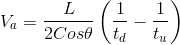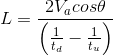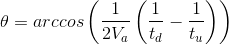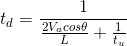# Acoustic Flowmeter Calculation

The calculator can calculate the average axial velocity of water-flow and other properties for selected values.
I want to calculate
 Acoustic Path Length between Transducer Faces(L) = m Angle between Acoustic Path and the Pipe's Longitudinal Axis(θ) = radian Acoustic Signal Downstream Travel Time(td) = s Acoustic Signal Upstream Travel Time(tu) = s Average Axial Velocity of Water Flow(Va) = m/s

The calculator can calculate the average axial velocity of water-flow or acoustic path length between transducer Faces or angle between acoustic path and the pipe's longitudinal axis or acoustic signal downstream travel time or acoustic signal upstream travel time as per selection based on appropriate given values.
Average Axial Velocity of Water Flow:Acoustic Path Length between Transducer Faces:Angle between Acoustic Path and the Pipe's Longitudinal Axis:Acoustic Signal Downstream Travel Time:Acoustic Signal Upstream Travel Time:where,

Va = Average Axial Velocity of Water Flow,

L = Acoustic Path Length between Transducer Faces,

θ = Angle between Acoustic Path and the Pipe's Longitudinal Axis,

td = Acoustic Signal Downstream Travel Time,

tu = Acoustic Signal Upstream Travel Time.